0%

## 一层神经网络：感知机与逻辑回归

#### M-P神经元模型

1943年，McCulloch和Pitts提出了沿用至今的M-P神经元。在这个模型中，神经元接收来自其他$M$个神经元传递过来的输入信号$x^{(j)},j=1,2,\cdots,M$， 这些输入信号通过带权重$\omega_j$的连接进行传递，神经元接收到总输入值与神经元的阈值进行比较，然后通过激活函数处理以产生神经元的输出。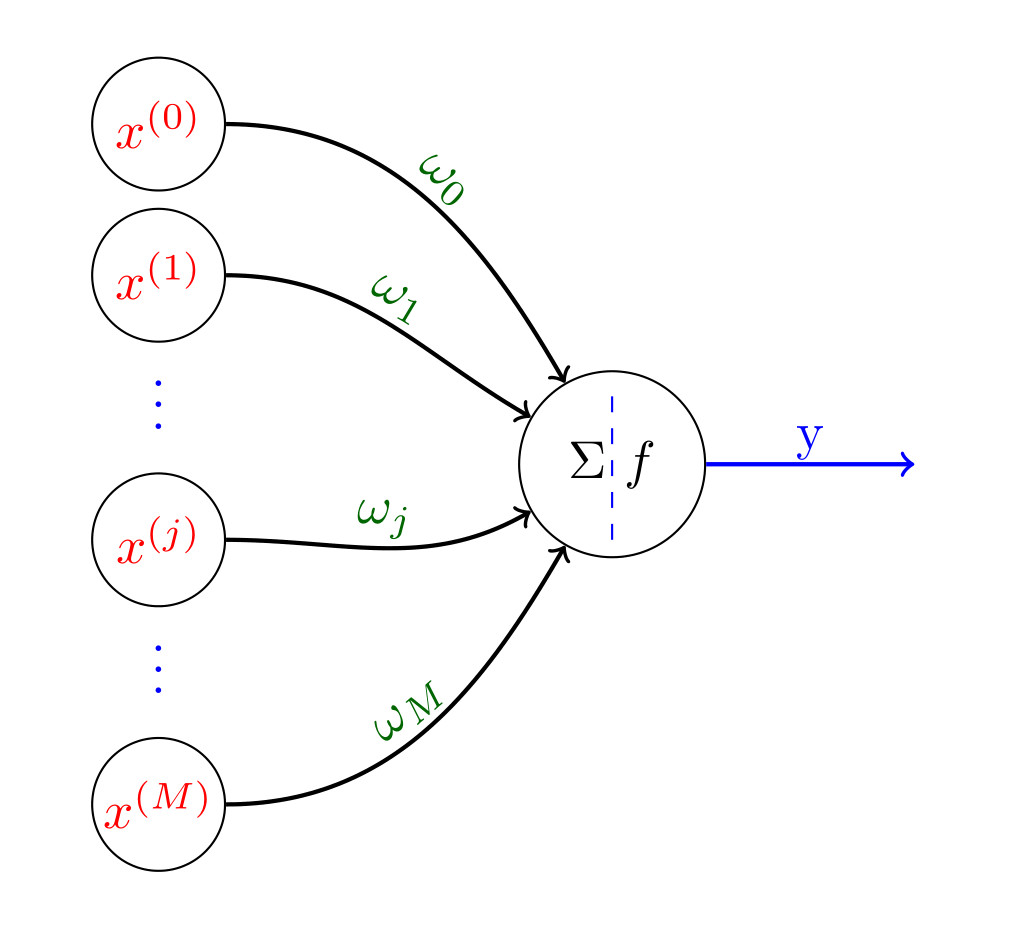图1

M-P神经元的数学表达式也可以表示如下：

#### 感知机与逻辑回归

• 激活函数：感知机使用的是阶跃函数，逻辑回归使用的Sigmoid函数，如图2所示：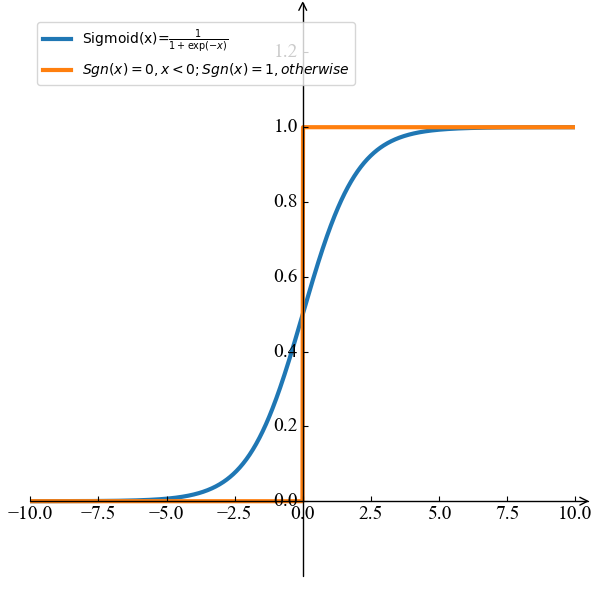图2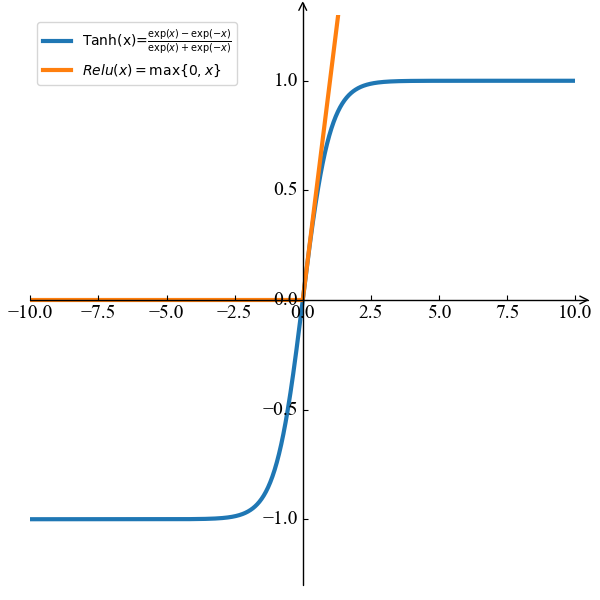图3

同时，最近比较流行的激活函数还有$tanh$ 和 $Relu$ 函数，如图3所示。

• 损失函数：感知机使用的是错误分类点到分类超平面距离最小化，逻辑回归使用的交叉熵最小化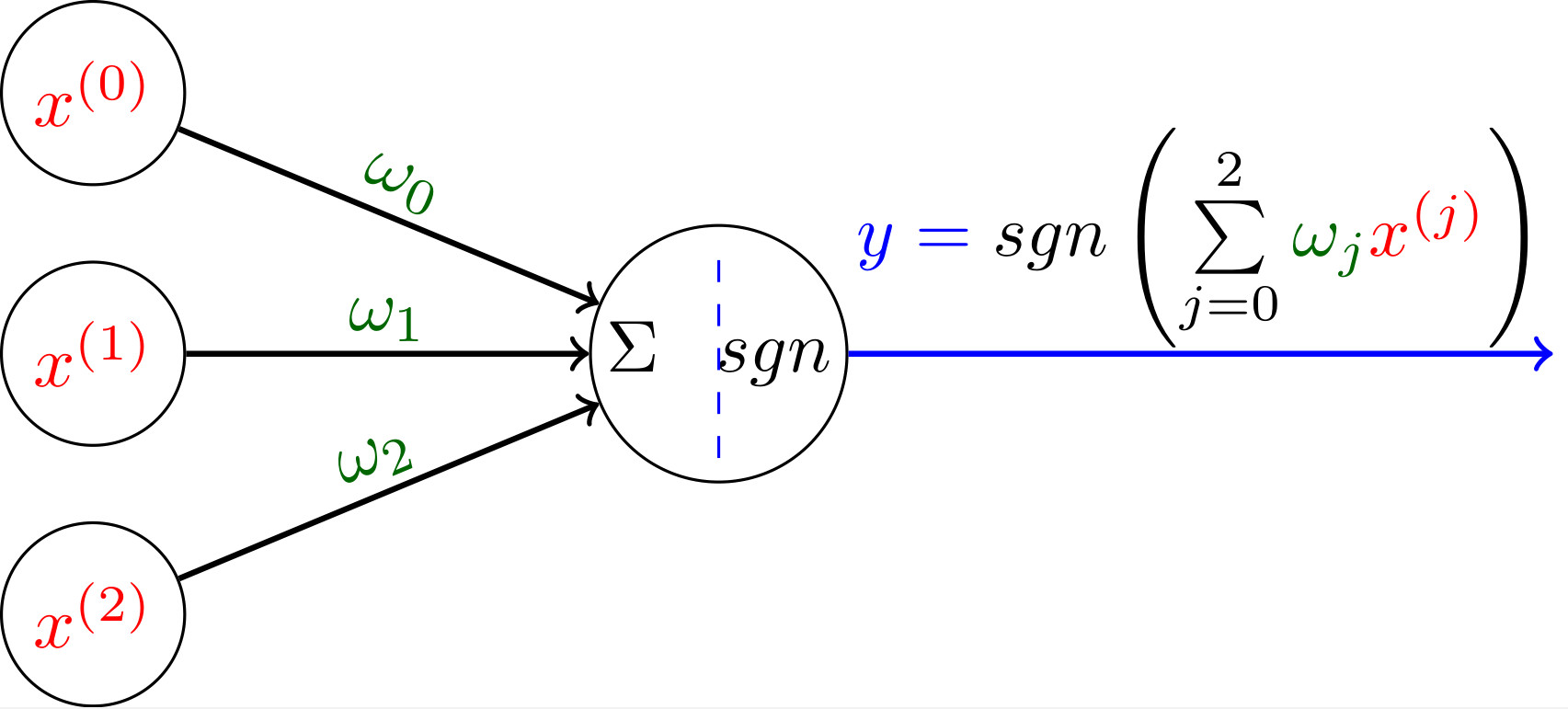图4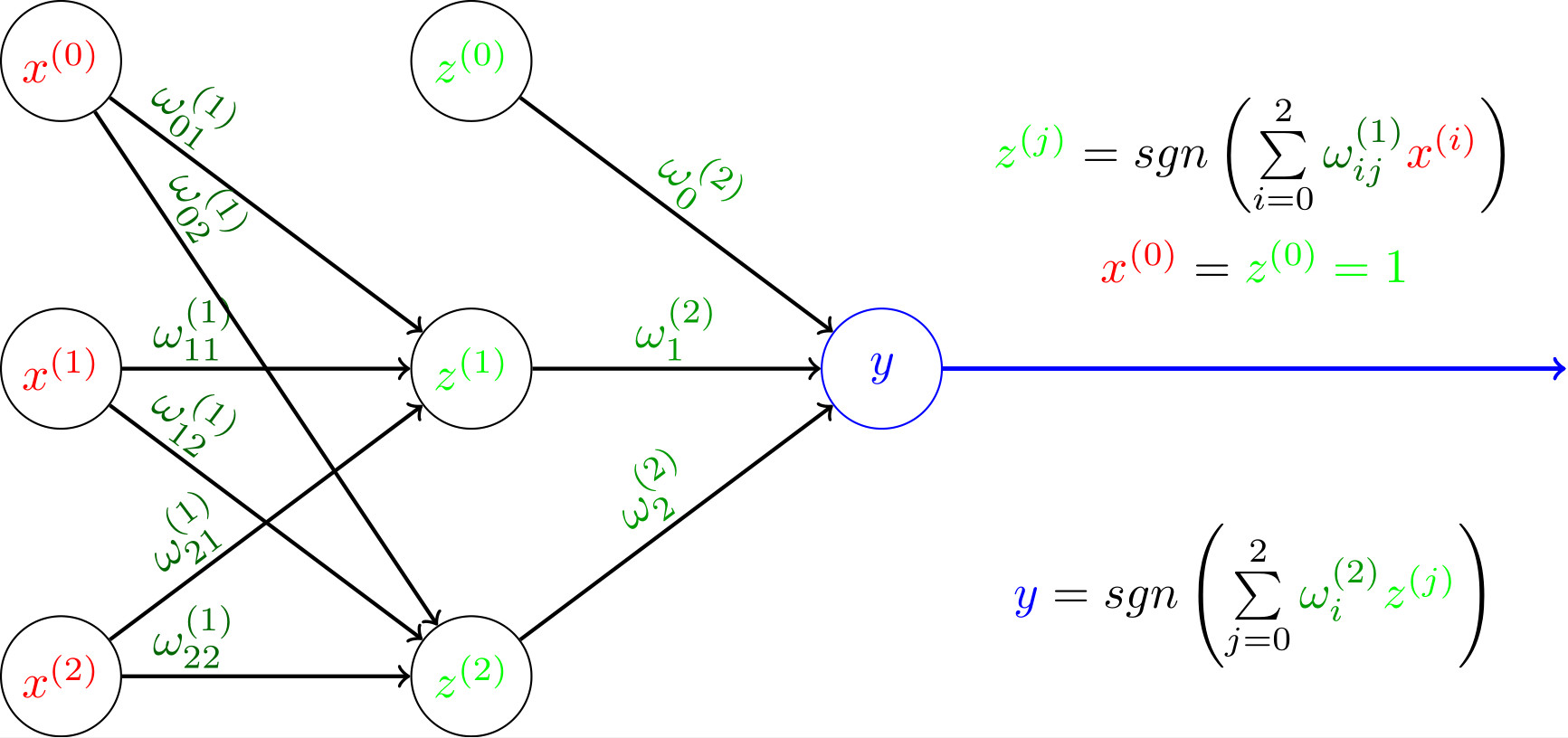图5

• 与计算 ($x^{(1)} \land x^{(2)}$)：令$\omega_1=\omega_2=1,\omega_0=-2$，我们有$y=sgn(x^{(1)}+x^{(2)}-2)$。此时，只有当$x^{(1)}=x^{(2)}=1$时，$y=1$
• 或计算 ($x^{(1)} \lor x^{(2)}$)：令$\omega_1=\omega_2=1,\omega_0=-0.5$，我们有$y=sgn(x^{(1)}+x^{(2)}-0.5)$。此时，当$x^{(1)}=1$或者 $x^{(2)}=1$时，$y=1$
• 非计算 ($\lnot x^{(1)}$)：令$\omega_1=-0.6$, $\omega_2=0,\omega_0=0.5$，我们有$y=sgn(-0.6x^{(1)}+0.5)$。此时，当$x^{(1)}=0$时，$y=1$; 当$x^{(1)}=1$时，$y=0$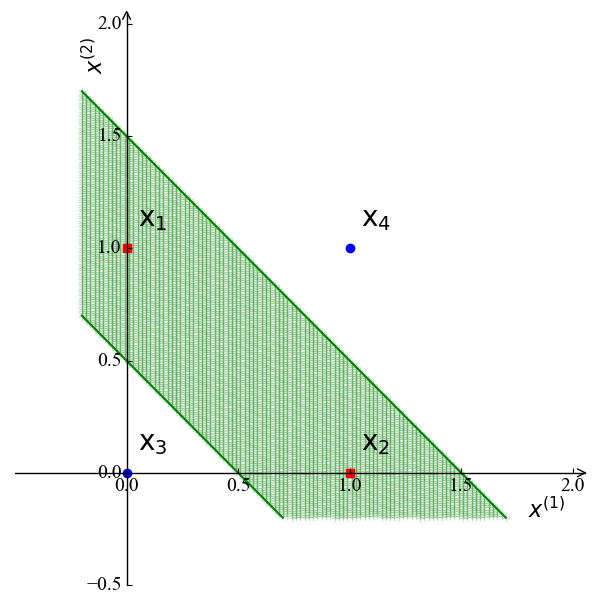图6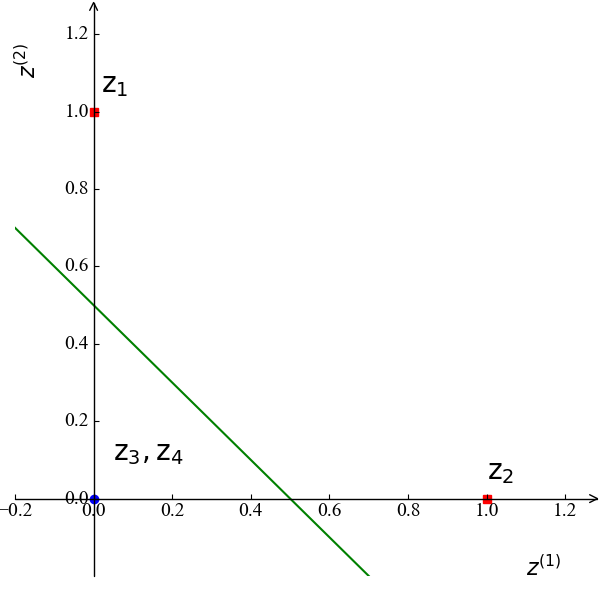图7

## 两层神经网络—BP 神经网络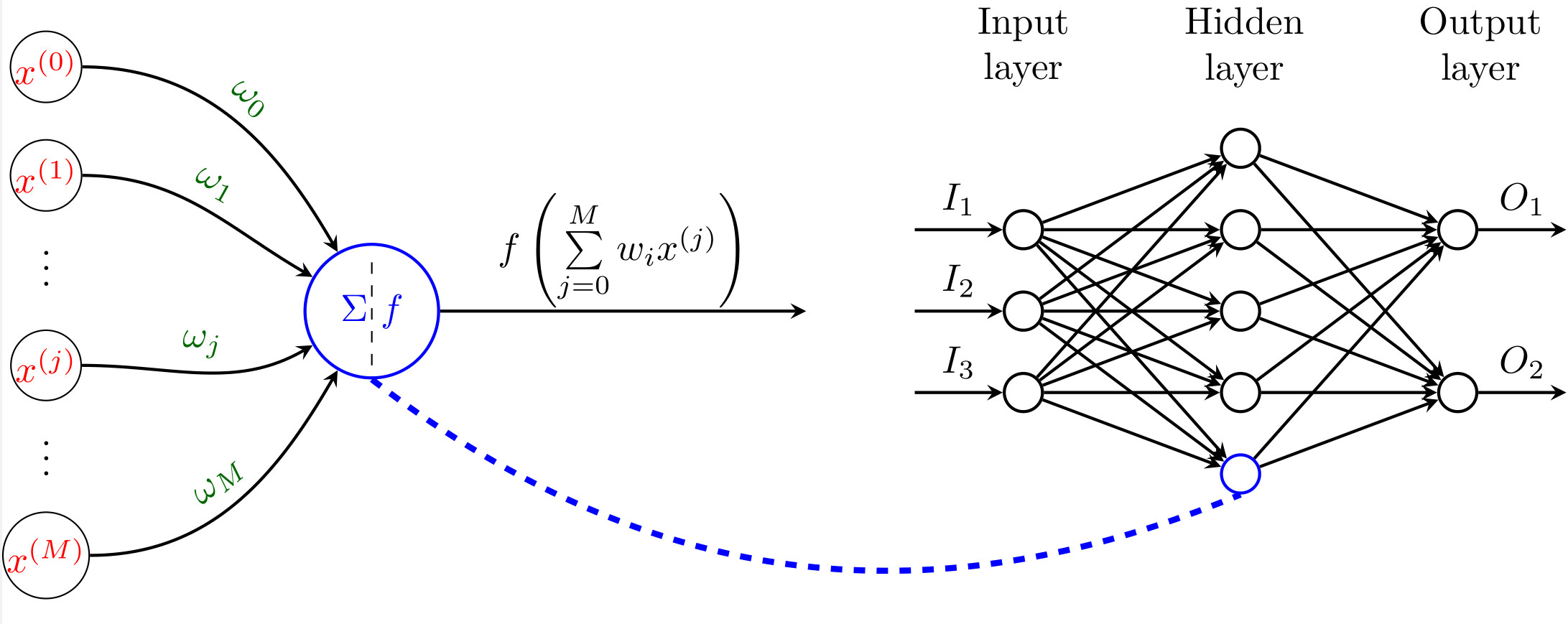图8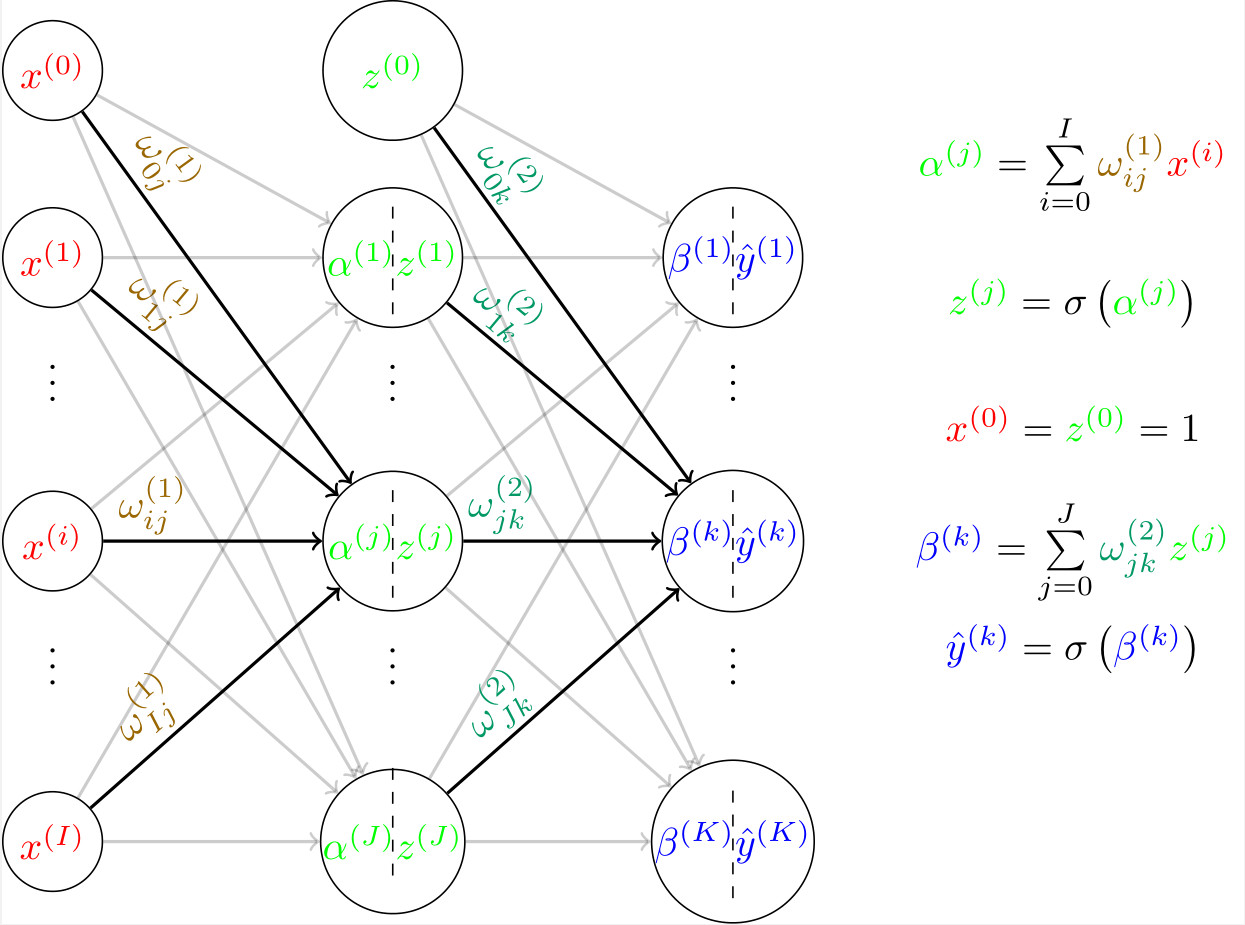图9

#### 误差反向传播

BP算法的核心就是误差反向传播。这里我们定义一个输入样例$\mathrm x$对应的误差为:

BP算法的目的是优化权重$\omega_{ij}^{(1)},\omega_{jk}^{(2)}$来最小化误差$E$。为此，BP算法采用了和梯度下降法一样的思想，以误差对权重的负梯度方向来不断更新权重，降低误差$E$。为此，我们需要计算输出层和隐藏层的$\frac{\partial E}{\partial\omega_{ij}^{(1)}}$和$\frac{\partial E}{\partial\omega_{jk}^{(2)}}$。